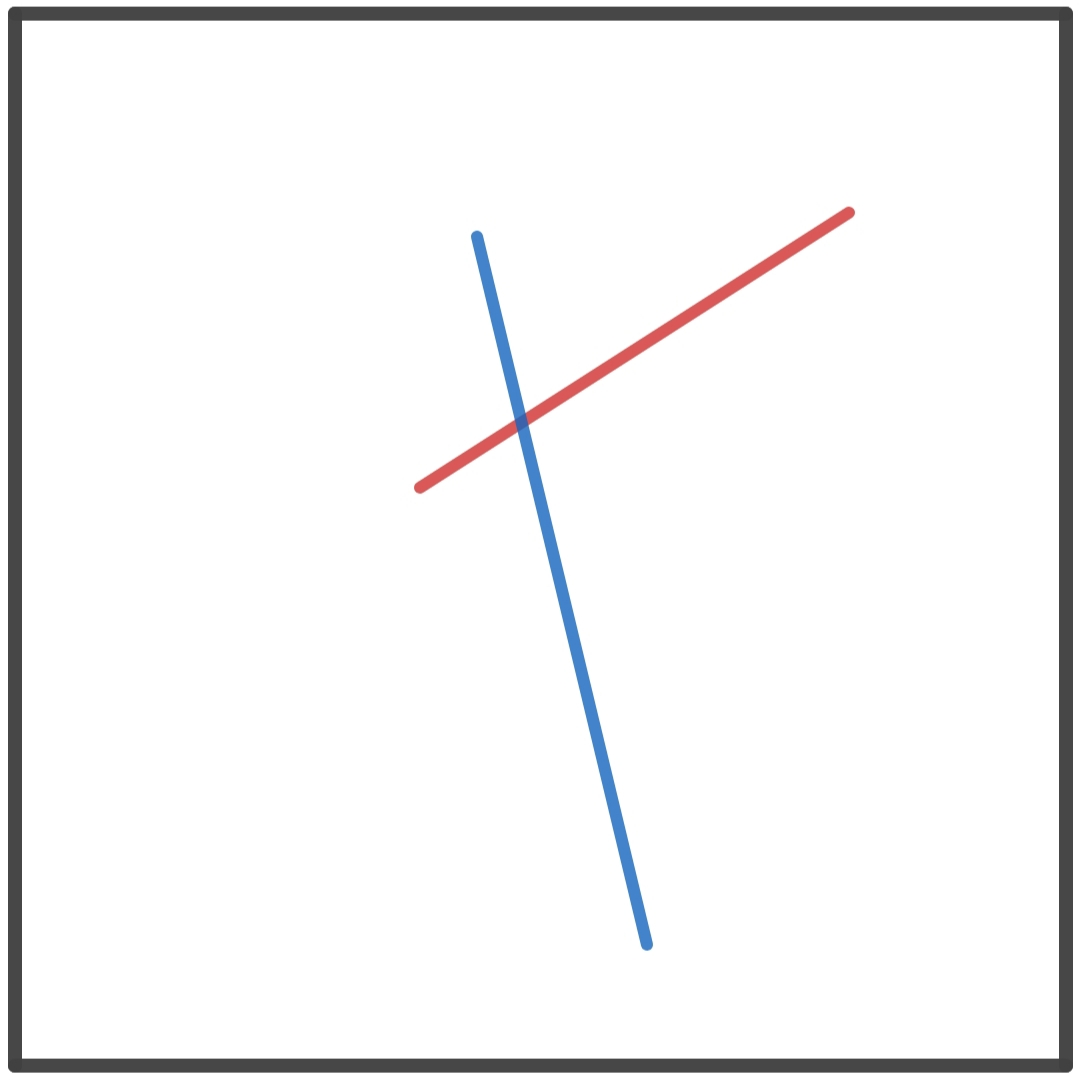# Doodle Probability

Probability Level 4Two points are randomly selected on a square sheet of paper and are joined to form a line segment.

This process is repeated to obtain another line segment.

What is the probability that the two lines intersect?

×